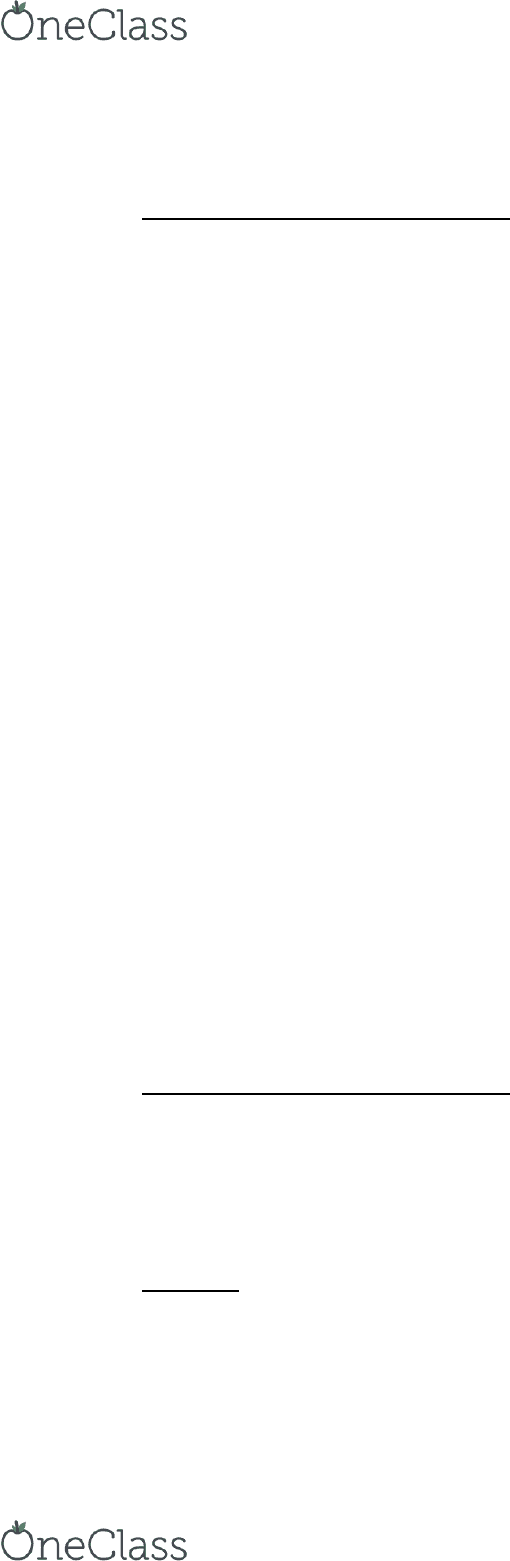# INFO 2020 Lecture 1: Week 1-3 Notes

42 views3 pagesWeek 1-3 Notes
Regression 1 (Y=Profit, X=The Rest)
R = correlation coefficient -1 to 1
R Square = coefficient of determination 0 to 1. This is the % of the variation
on the DV known from variation on the IV's
RHO stands for R
if p<.05 then reject
Ho
Ho means R = 0
Significance F = the p value
Ha means R doesn’t = 0
Because R doesn’t
= 0,
there is a
correlation
not a useful variable for us
this is a good
model
cannot tell coefficient from 0
Coefficient column is important
one
Profit = intercept + coefficients (categories)
EX: Bed room coefficient =
\$6,000
if we incr. by 1 bed room, profit goes up \$6,000
P-Value column does individual hypothesis tests on each variable
If coefficient and Lower 95% level are both negative OR both
positive, then good
If one of each, then bad
Regression 2 (Y=Profit, X=The Rest)
R and R Square are both high =
GOOD
Significance F (P value) very low
= GOOD
P-Values
If large, then fail to reject the hypothesis
If small
find more resources at oneclass.com
find more resources at oneclass.com
Unlock document

This preview shows page 1 of the document.
Unlock all 3 pages and 3 million more documents.